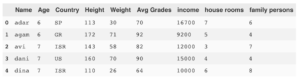Pandas is a great python library for data handling. It declares 2 main classes:

1. Series – for one dimensional array
2. DataFrame – for 2 dimensional tables (and with multi-indexing can display more dimensions)

Typical flow of using Pandas will be – load the data, manipulate and store again. This is very similar to SQL use with Select, Insert, Update and Delete statement

In this post i will cover the basic operations in pandas compared to SQL statements

I will use a simple CSV file, load it to a  dataframe and run all the commands on it:

```import numpy as np
import pandas as pd## Select

Select * from df where index between 2 and 6

`df[2:6]`

Select * from df where (index between 3 and 9) and (index % 2 = 1)

`df[3:9:2]`

Select Name from df

`df['Name']`

Select Name, Age from df

`df[['Name','Age']]`

Select Name where index between 2 and 6

`df['Name'][2:6]`

Select Name,Age where index between 2 and 6

`df[['Name','Age']][2:6]`

Note for reference – Same operations with loc:

```df.loc # index 2 as series
df.loc[2:2] # index 2 as dataframe
df.loc[2:6] # indexes 2-6
df.loc[2:6:2] # even indexes 2-6
df.loc[2:2]['Age'] # Age where index=2
df.loc[1,'Age'] # Age where index=1
df.loc[1:13:2,'Age':'income':2] # odd rows from 1 to 13 and even cols from Age to income```

Same operations with iloc:

```df.iloc # where index=1
df.iloc[1,1] # Age where index=1
df.iloc[1:4] # where index between 1 and 3
df.iloc[1:4,2:4] # Country, Height where index between 1 and 3```

Select * from df where Age > 12

`df[df['Age']>10]`

Select Name, Country  from df where Age > 12

`df[df['Age']>10][['Name','Country']]`

Select * from df where Age>12 or Height > 130

`df[(df['Age']>12) | (df['Height'] > 130)]`

Select * from df where Age>12 and Height > 130

`df[(df['Age']>12) & (df['Height'] > 130)]`

## Insert

Insert into df values (‘eli’,4,’DF’,100,20,20,2000,4,4)

`df.loc[df.index.size]=['eli',4,'DF',100,20,20,2000,4,4]`

Insert into df select * from df2

`df.append(df2)`

Insert new column with values (alter table df add Inc int; update df set Inc=income*2)

`df['Inc']=df['income']*2`

## Update

Update df set Age=30

`df.loc[:,'Age']=30`

Update df set Age=20 where income>20000

`df.loc[df['income']>20000,'Age']=20`

Update df set income=income*2

`df['income']*=2`

Complex Update using iterator:

```for i,v in df.iterrows():
if v.Age > 10:
df.loc[i,'Weight'] = 888
```

The above is a simple example but you can add as much logic as you need to the loop

## Delete

Delete df where index=6

`df.drop(6)`

Delete df where Age=6

`df.drop(df['Age']==6,inplace=True)`

Delete column (alter table drop column Age)

```df.drop('Age',axis=1)
```

## Aggregates and Group By

Select count(*) from df

`df.agg('count')`

Select count(*),max(*) from df

`df.agg(['count','max'])`

Select count(Name), sum(income) , max(Age) from df

`df.agg({'Name':'count', 'income':'sum' , 'Age':'max'})`

select Country,count(*) from df group by Country

`df.groupby('Country').count()`

select Country,[family persons],count(*) from df group by Country,[family persons]

`df1=df.groupby(['Country','family persons']).count()`

Tagged ,